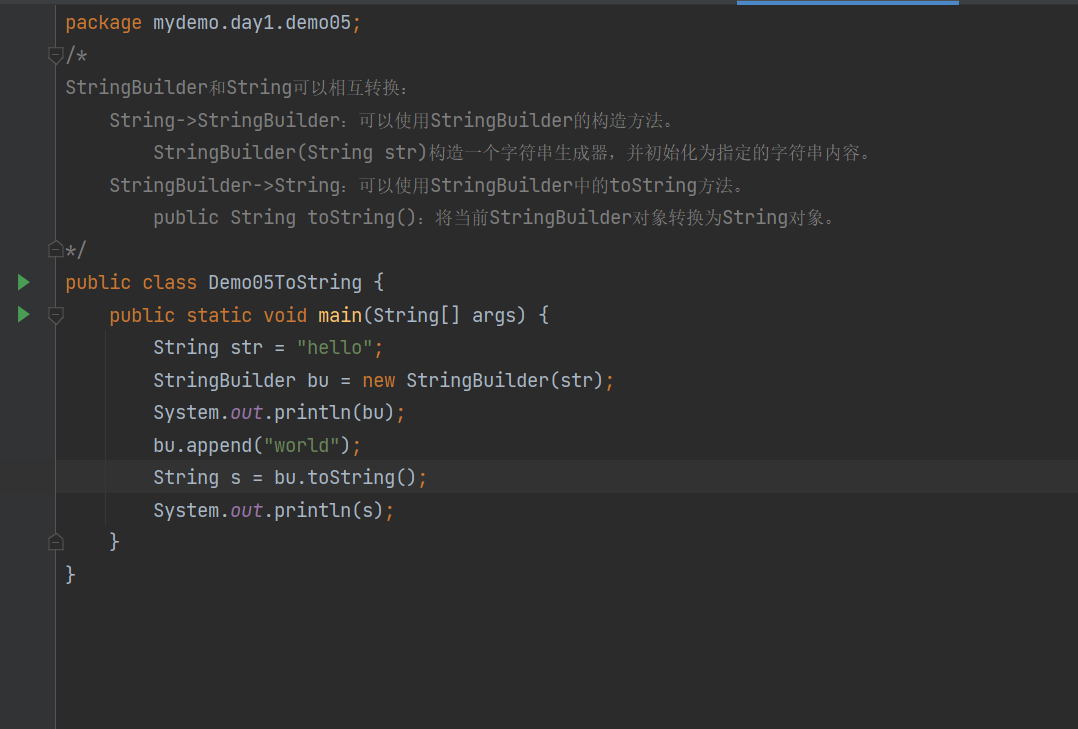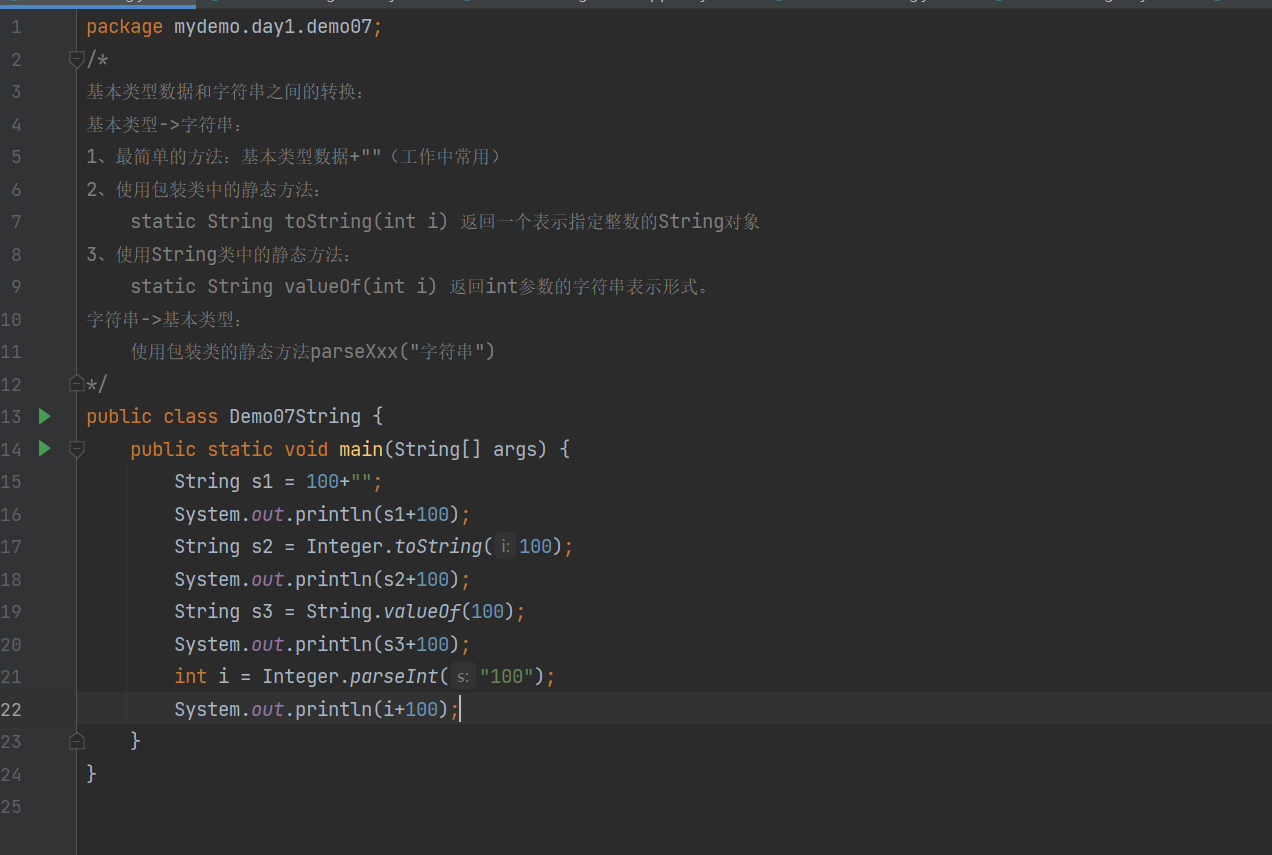课堂笔记记录2311-杨同学

热门标签/*

Integer(int value) 构造一个新分配的 Integer 对象，它表示指定的 int 值。
Integer(String s) 构造一个新分配的 Integer 对象，它表示 String 参数所指示的 int 值。

static Integer valueOf(int i) 返回一个表示指定的 int 值的 Integer 实例。
static Integer valueOf(String s) 返回保存指定的 String 的值的 Integer 对象。

int intValue() 以 int 类型返回该 Integer 的值。
*/
public class Demo06Integer1 {
public static void main(String[] args) {
//装箱
//构造方法
Integer in1 = new Integer(“100”);
System.out.println(in1);
Integer in2 = new Integer(1);
System.out.println(in2);
//静态方法
Integer in3 = Integer.valueOf(100);
System.out.println(in3);
Integer in4 = Integer.valueOf(“3”);
System.out.println(in4);
//拆箱
int a = in1.intValue();
int sum = a + 100;
System.out.println(sum);
}
}评论 抢沙发觉得文章有用就打赏一下文章作者

支付宝扫一扫打赏微信扫一扫打赏Vieu3.3主题Q Q 登 录